# Grammar, regular

A context-free grammar (cf. Grammar, context-free) each rule of which has the form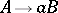or, whereandare non-terminal symbols andis one of the terminal symbols. (Rules of the form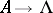, where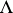is the empty string, are also sometimes allowed; the class of languages generated is then extended only at the expense of the languages obtained by adding the stringto the previous languages.) For each regular grammar it is possible to construct a finite automaton (cf. Automaton, finite) equivalent to it. The class of languages generated by regular grammars (regular or automatic languages) coincides with the class of regular sets if rules with an empty right-hand side are allowed. The regular languages form a proper subclass of the class of linear languages (cf. Grammar, linear). Thus, the linear language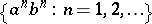is not regular. The class of regular languages is closed under union, intersection, concatenation, substitution, and truncated iteration (and, in the presence of rules with an empty right-hand side, also with respect to iteration). The intersection of a context-free language with a regular language is again a context-free language.
A regular grammar is usually illustrated by a transition diagram — an oriented multi-graph whose vertices are non-terminal symbols, and a vertexis connected to a vertexby means of an arc, marked with the (terminal) symbol, ifis a rule of the grammar; in addition, the transition diagram contains one more terminal vertex, connected with a non-terminal symbolby means of an arc marked by the symbolifis a rule of the regular grammar. (If there are rules of the form, each symbolgoverned by this rule is also considered to be a terminal vertex.)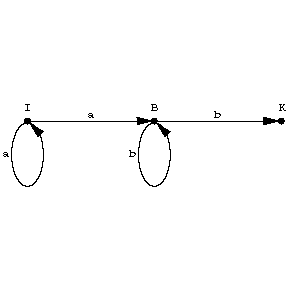A string in the basic vocabulary is derivable in the grammar from a non-terminal symbolif and only if it is "written down" in some path in the diagram going fromto the terminal vertex. The figure represents a transition diagram of a regular grammar with the rules,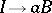,,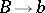(is the initial symbol,is the terminal vertex), which generates the language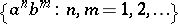.